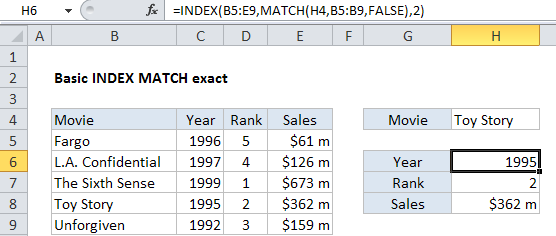## Excel Office

Excel How Tos, Tutorials, Tips & Tricks, Shortcuts

# Basic INDEX MATCH exact in Excel

This tutorial shows how to calculate Basic INDEX MATCH exact in Excel using the example below;

## Formula

```=INDEX(data,MATCH(value,lookup_column,FALSE),column)```

## Explanation

This example shows how to use INDEX and MATCH to get information from a table based on an exact match. In the example shown, the formula in cell H6 is:

`=INDEX(B5:E9,MATCH(H4,B5:B9,FALSE),2)`

which returns 1995, the year the movie Toy Story was released.

### How this formula works

This formula uses MATCH to get the row position of Toy Story in the table, and INDEX to retrieve the value at that row in column 2.

Worked Example:   Get nth match with INDEX / MATCH in Excel

MATCH is configured to look for the value in H4 in column B:

`MATCH(H4,B5:B9,FALSE)`

Note that the last argument is FALSE, which forces MATCH to perform an exact match.

MATCH finds “Toy Story” on row 4 and returns this number to INDEX as the row number. INDEX is configured with an array that includes all the data in the table, and the column number is hard-coded as 2. Once MATCH returns 4 we have:

`=INDEX(B5:E9,4,2)`

INDEX then retrieves the value at the intersection of the 4th row and 2nd column in the array, which is “1995”.

Worked Example:   Position of max value in list in Excel

The other formulas in the example are the same except for the column number:

```=INDEX(B5:E9,MATCH(H4,B5:B9,FALSE),2) // year
=INDEX(B5:E9,MATCH(H4,B5:B9,FALSE),3) // rank
=INDEX(B5:E9,MATCH(H4,B5:B9,FALSE),4) // sales```

### INDEX with a single column

In the example above, INDEX receives an array that contains all data in the table. However, you can easily rewrite the formulas to work with one column only, which eliminates the need to supply a column number:

```=INDEX(C5:C9,MATCH(H4,B5:B9,FALSE)) // year
=INDEX(D5:D9,MATCH(H4,B5:B9,FALSE)) // rank
=INDEX(E5:E9,MATCH(H4,B5:B9,FALSE)) // sales```

In each case, INDEX receives an single-column array that corresponds to the data being retrieved, and MATCH supplies the row number.

Worked Example:   Partial match against numbers with wildcard in Excel